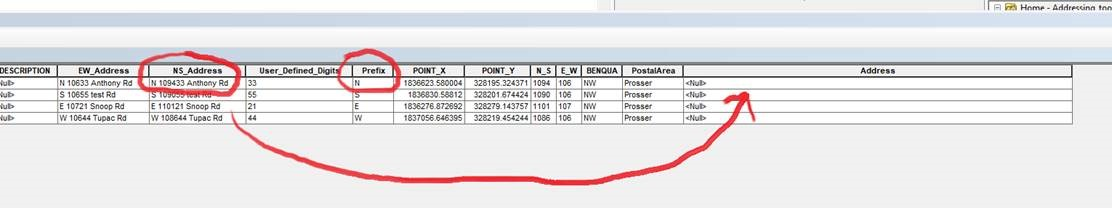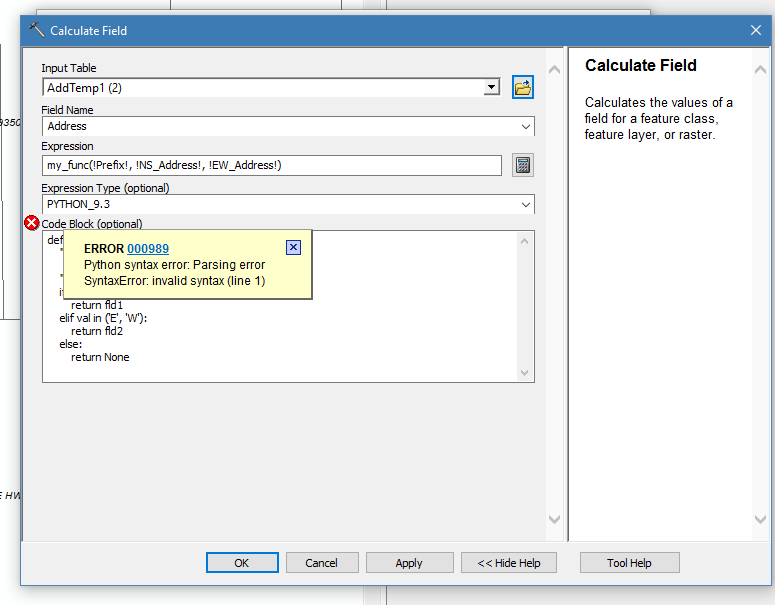666
6
06-19-2018 08:53 AMbyMVP

I need some assistance creating a ArcPy script to field calculate and display the value of a field based on the input of another field. I would like to show the value of attribute NS_Address if the Prefix attribute is N or S and I would like to show the value of attribute EW_Address if the Prefix attribute is E or W.1 Solution

Accepted SolutionsbyMVP Esteemed Contributor

put the function in the code block.

the expression in the expression box

and calculate away

``````def my_func(val, fld1, fld2)
""" NS_Address if the Prefix attribute is N or S
EW_Address if the Prefix attribute is E or W
"""
if val in ('N', 'S'):
return fld1
elif val in ('E', 'W'):
return fld2
else:
return None‍‍‍‍‍‍‍‍‍‍‍‍‍‍‍‍‍‍‍‍``````

expression

6 RepliesbyMVP Regular Contributor

Something like this? Untested code...

```import arcpy
feature_class_path =r"xxxx"

for row in cursor:
row_parts = row.split(" ")
prefix = ""
if row_parts == "N" or row_parts == "S":
else:
row = prefix + " " + row_parts + " " + row_parts
cursor.updateRow(row)```byMVP Esteemed Contributor

put the function in the code block.

the expression in the expression box

and calculate away

``````def my_func(val, fld1, fld2)
""" NS_Address if the Prefix attribute is N or S
EW_Address if the Prefix attribute is E or W
"""
if val in ('N', 'S'):
return fld1
elif val in ('E', 'W'):
return fld2
else:
return None‍‍‍‍‍‍‍‍‍‍‍‍‍‍‍‍‍‍‍‍``````

expressionbyMVP

Dan,

Thanks for the reply. I received the error below when attempting to run that calculation.byMVP

I just had to add a colon after the first line. The expression ran perfectly! Thank you!!!byMVP Esteemed Contributor

Anthony, no problem

You should close the question so others can find the answerbyMVP Esteemed Contributor

I suppose